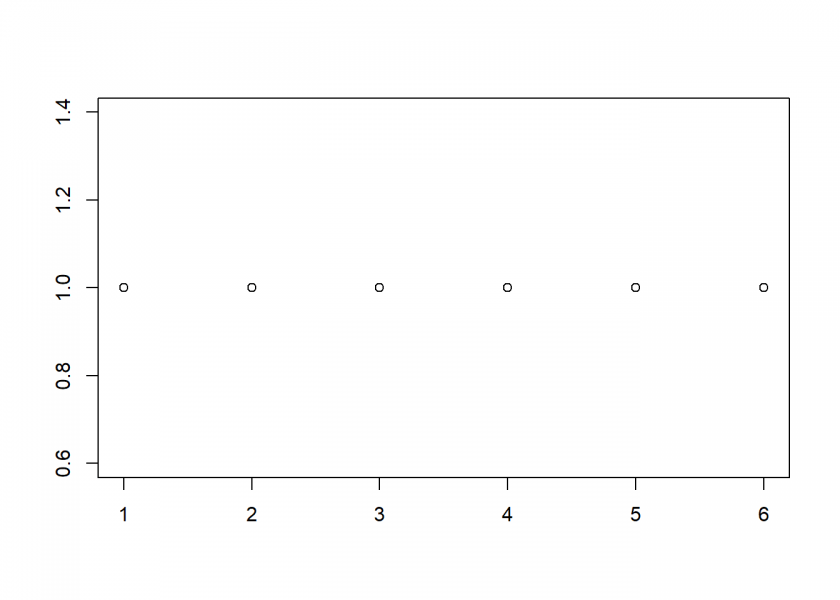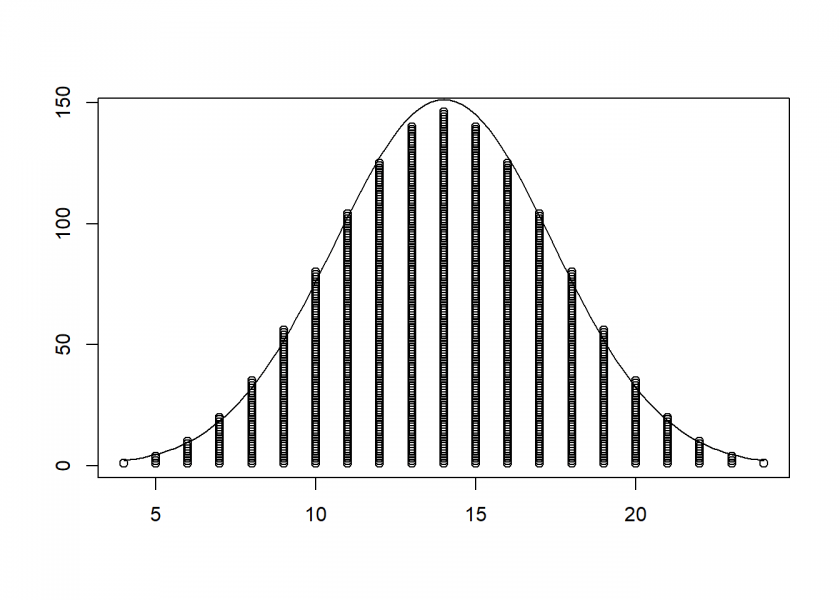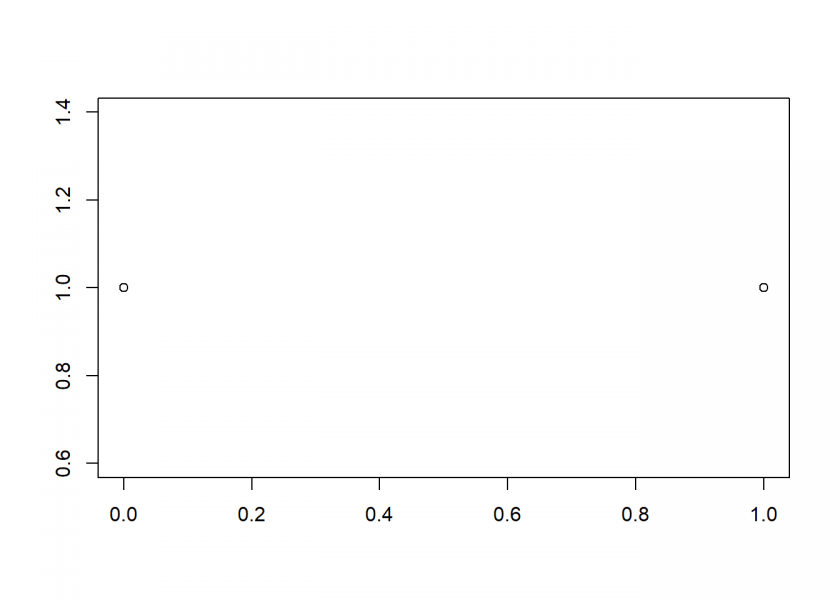Want to share your content on R-bloggers? click here if you have a blog, or here if you don't.How can the Normal Distribution arise out of a completely symmetric set-up? The so-called Central Limit Theorem (CLT) is a fascinating example that demonstrates such behaviour. If you want to get some intuition on what lies at the core of many statistical tests, read on!

The Central Limit Theorem (CLT) states:

The sum (or the average = scaled sum) of a big number of independent and identically distributed (i.i.d.) random variables with defined (= finite variance) are nearly normally distributed. This constitutes the special status of the Normal Distribution (= bell curve or Gaussian).

What are i.i.d. random variables? A few years ago I gave the following explanation on Cross Validated:

A good example is a succession of throws of a fair coin: The coin has no memory, so all the throws are “independent”. And every throw is 50:50 (heads:tails), so the coin is and stays fair – the distribution from which every throw is drawn, so to speak, is and stays the same: “identically distributed”.

As an example, let us start with a simple fair die, which gives us a perfectly symmetric uniform (discrete) distribution form 1 to 6:

```plot_hist <- function(x) {
plot(sort(x), sequence(table(x)), xlab = "", ylab = "")
}

X <- 1:6 # one die
plot_hist(X)
```Or as a frequency table:

```table(X)
## X
## 1 2 3 4 5 6
## 1 1 1 1 1 1
```

Now, we either throw this die twice and add the dots or we take two dice, again adding their dots. The range obviously lies between 2 and 12, but not all of those outcomes are created equal:

```X2 <- rowSums(expand.grid(1:6, 1:6))
plot_hist(X2)
```Again as a frequency table:

```table(X2)
## X2
##  2  3  4  5  6  7  8  9 10 11 12
##  1  2  3  4  5  6  5  4  3  2  1
```

We can see that 2 and 12 only have one possibility of happening (1+1 and 6+6), whereas 7 has six different combinations (1+6, 2+5, 3+4, 4+3, 5+2 and 6+1).

Here we are at the center of the symmetry-break: we have two perfectly symmetric entities and by combining them we get a triangle-like structure. Why? Basically this is what is happening:

Mathematically this is called a convolution: by summing all possible combinations you are sliding the original uniform distribution over itself. This naturally produces less overlap (= sum) at the edges and maximal overlap at the center!

You can continue in this manner, taking the sum of three dice…

```X3 <- rowSums(expand.grid(1:6, 1:6, 1:6))
plot_hist(X3)
```…and four dice, superimposing a fitted normal distribution:

```X4 <- rowSums(expand.grid(1:6, 1:6, 1:6, 1:6))
plot_hist(X4)
curve(dnorm(x, mean = mean(X4), sd = sd(X4)) * length(X4), add = TRUE)
```You can see that the resulting structure resembles the normal distribution more and more. Seen this way the Normal Distribution is the honed triangle from above!

Because there are many processes in real life that act additively (like e.g. growth processes) the Normal Distribution holds a special place in many real-world phenomena (e.g. heights). This is the reason why it constitutes the basis for many statistical tests in inferential (= inductive) statistics (see also From Coin Tosses to p-Hacking: Make Statistics Significant Again!).

The go even more minimalistic, we can even see this symmetry-break with said coin tosses:

```X <- 0:1 # one coin
plot_hist(X)
``````X2 <- rowSums(expand.grid(0:1, 0:1))
plot_hist(X2)
```To answer our starting question: in the end, it is the process, or more exactly, the mathematical operation that we use (sliding over the outcomes of our random experiments) which gives us a honed version of a triangle: the Normal Distribution!

Yet the derivation of the Paranormal Distribution remains a mystery…